## DC battery current vs AC phase current on a triphase converter

• Titre : Courant de batterie DC vs courant de phase AC sur un convertisseur triphasé
• Auteur : Thierry LEQUEU
• Date : 26 mars 2023

The purpose here is to express a simple relationship between the DC current from the battery and the phase current in a three-phase motor speed controller.

On the battery side, the electrical input power of the controller is given by :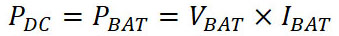On the motor side, the electrical output power of the controller is given by :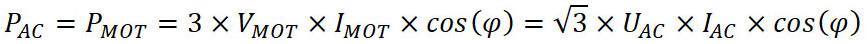With IAC the rms line current (in one of the 3 phases) of the motor and UAC the rms voltage between 2 phases of the motor.

For a SEVCON GEN4 controller, the efficiency value is about 90%: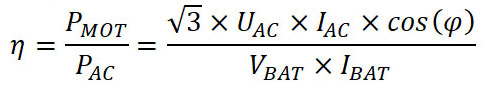The relationship between the DC current of the battery and the phase current of the motor is given by: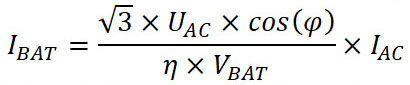For a SEVCON GEN4 controller, the relationship between UAC the rms voltage between 2 phases of the motor and VBAT the DC voltage of the battery is given by: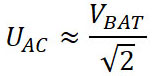The relationship between the battery current and the phase current then becomes ::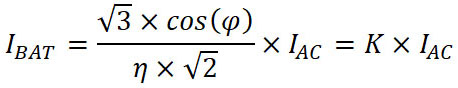With cos(phi) = 0.7 and efficiency = 90%, the value K = 0.953